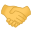# C/C++C atan2 and floating point

#### AlexanderProphet

##### Active Member

Reaction score: 35
Messages: 156

Hi,
I've noticed that when I pass values very close to zero to atan2 it still gives an angle of +/- 180 degrees or +/- 90 degrees. But KDE's calculator's atan gives zero for the same inputs. Also the book I am reading (Understanding Digital Signal Processing) gives zeroes in the example I am working through.
I'm guessing these are floating point errors? Though I am surprised because I'm using double precision floats.
I made a hack "atan2" where I test for both values having a magnitude below 0.001 and it works (gives the same answers as the book) - however it is just that: a hack that I'm concerned may come back to bite me.
Any thoughts or suggestions?

#### shkhln

##### Aspiring Daemon

Reaction score: 225
Messages: 702

I've noticed that when I pass values very close to zero to atan2 it still gives an angle of +/- 180 degrees or +/- 90 degrees.
What does it matter? 0.001 isn't any less of a number than 1000.0.

But KDE's calculator's atan gives zero for the same inputs.
?

I'm guessing these are floating point errors?
No, those are a different thing altogether.

#### AlexanderProphet

##### Active Member

Reaction score: 35
Messages: 156

It matters greatly because the phase relationship of two sinusoids is zero in the book and 90 degrees on my computer. Due to some kind of numerical error involving very tiny values which admittedly may be my fault but I'm inclined to blame FP.
Can anyone else help?

#### aragats

##### Daemon

Reaction score: 520
Messages: 1,248

I've noticed that when I pass values very close to zero to atan2 it still gives an angle of +/- 180 degrees or +/- 90 degrees.
I really don't understand what's wrong with that. From atan2(3):
Code:
``````   atan2(y, x) :=    atan(y/x)                       if x > 0,
sign(y)*(pi - atan(|y/x|))      if x < 0,
0                               if x = y = 0, or
sign(y)*pi/2                    if x = 0 ≠ y.``````
Zero is only when both x and y are zeros. Am I missing anything?
Just tested:
Code:
``````% cat atan.c
#include <stdio.h>
#include <math.h>

int main()
{
double f1 = 0.00000001;
double f2 = 0.00000001;

printf ("atan2(%0.8f, %0.8f)=%f\n", f1, f2, atan2(f1, f2));
}

% cc -o atan -lm atan.c  && ./atan
atan2(0.00000001, 0.00000001)=0.785398``````

#### obsigna

##### Aspiring Daemon

Reaction score: 560
Messages: 957

I deleted my first response, because in the meantime I read the atan2(3) manual page on macOS 10.13, and this one knows much more special cases. Now the point is, that the results of the special cases of atan2 on FreeBSD actually resembles much more to the macOS man page than to the one of FreeBSD. Please compare atan2(3) with:
Code:
``````SPECIAL VALUES
atan2(+-0, -0) returns +-pi.
atan2(+-0, +0) returns +-0.
atan2(+-0, x) returns +-pi for x < 0.
atan2(+-0, x) returns +-0 for x > 0.
atan2(y, +-0) returns +pi/2 for y > 0.
atan2(y, +-0) returns -pi/2 for y < 0.
atan2(+-y, -infinity) returns +-pi for finite y > 0.
atan2(+-y, +infinity) returns +-0 for finite y > 0.
atan2(+-infinity, x) returns +-pi/2 for finite x.
atan2(+-infinity, -infinity) returns +-3*pi/4.
atan2(+-infinity, +infinity) returns +-pi/4.``````
Perhaps a PR should be submitted asking for corrections of the atan2(3) man page.

•aragats

#### aragats

##### Daemon

Reaction score: 520
Messages: 1,248

Code:
``````SPECIAL VALUES
atan2(+-0, -0) returns +-pi.
atan2(+-0, +0) returns +-0.``````
Yes, it does – just checked. The man page needs to be updated, but how it's related to the OP's question?
«Close to zero» – is not zero.

#### obsigna

##### Aspiring Daemon

Reaction score: 560
Messages: 957

Agreed, the OP’s report is not very conclusive. His values ±180 and ±90 degrees, i.e. ±3.141593... and ±1.570796... would actually be the result of atan2(±0.0, -0.001) = ±3.141593 and atan2(±0.001, 0.0) = 1.570796. However, it is not quite clear what he is talking about.

#### aragats

##### Daemon

Reaction score: 520
Messages: 1,248

It properly works down to the allowed minimum when the compiler spits out a warning:
Code:
``````warning: magnitude of floating-point constant too small for type 'double'; minimum is
4.9406564584124654E-324 [-Wliteral-range]
double f2 = -1e-324;``````
At that point it prints:
Code:
``atan2(0, -0)=3.141593``
With larger numbers it calculates the actual value:
Code:
``atan2(1e-300, -1e-300)=2.356194``

#### Eric A. Borisch

##### Well-Known Member

Reaction score: 246
Messages: 421

When in doubt, check the source. See also.

KDE's calculator is likely "fixing" or "cleaning" values that are close to +-0 to +0. And as for your code, it depends on what you mean by very close to zero.

All floating point is in error; it's just a question by how much...#### AlexanderProphet

##### Active Member

Reaction score: 35
Messages: 156

Thanks everyone for your replies. Sorry for the delay in getting back to you. Yes I formulated the problem very badly. I think what is happening is not due to atan/atan2, but due to the fact that my double precision value has gone through a lot of processing before being passed to atan/atan2. And also post-processing - conversion to degrees.
One day I'm going to post something sensible on here...